## ML Aggarwal Class 10 Solutions for ICSE Maths Chapter 11 Section Formula Ex 11

These Solutions are part of ML Aggarwal Class 10 Solutions for ICSE Maths. Here we have given ML Aggarwal Class 10 Solutions for ICSE Maths Chapter 11 Section Formula Ex 11

More Exercises

Midpoint Calculator is used to find the midpoint between 2 line segments using the midpoint formula. Use our calculator to find accurate midpoints step by step.

Question 1.
Find the co-ordinates of the mid-point of the line segments joining the following pairs of points:
(i) (2, – 3), ( – 6, 7)
(ii) (5, – 11), (4, 3)
(iii) (a + 3, 5b), (2a – 1, 3b + 4)
Solution:
(i) Co-ordinates of the mid-point of (2, -3), ( -6, 7)
$$\left( \frac { { x }_{ 1 }+{ x }_{ 2 } }{ 2 } ,\frac { { y }_{ 1 }+{ y }_{ 2 } }{ 2 } \right) or$$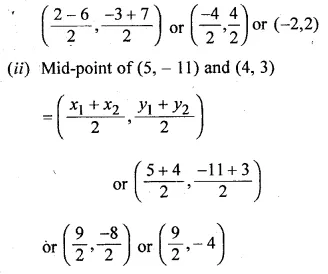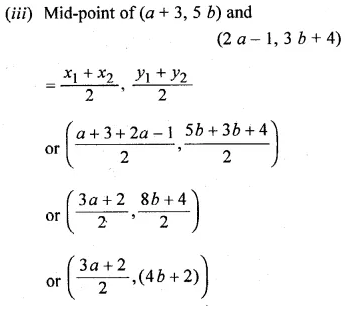Question 2.
The co-ordinates of two points A and B are ( – 3, 3) and (12, – 7) respectively. P is a point on the line segment AB such that AP : PB = 2 : 3. Find the co-ordinates of P.
Solution:
Points are A (-3, 3), B (12, -7)
Let P (x1,  y1) be the point which divides AB in the ratio of m1 : m2 i.e. 2 : 3
then co-ordinates of P will be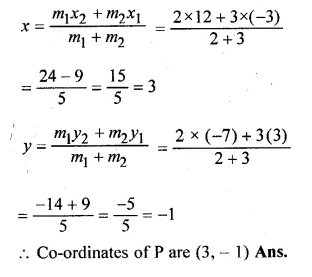Question 3.
P divides the distance between A ( – 2, 1) and B (1, 4) in the ratio of 2 : 1. Calculate the co-ordinates of the point P.
Solution:
Points are A (-2, 1) and B (1, 4) and
Let P (x, y) divides AB in the ratio of m1 : m2 i.e. 2 : 1
Co-ordinates of P will beQuestion 4.
(i) Find the co-ordinates of the points of trisection of the line segment joining the point (3, – 3) and (6, 9).
(ii) The line segment joining the points (3, – 4) and (1, 2) is trisected at the points P and Q. If the coordinates of P and Q are (p, – 2) and $$\left( \frac { 5 }{ 3 } ,q \right)$$ respectively, find the values of p and q.
Solution:
(i) Let P (x1, y1) and Q (x2, y2) be the points
which trisect the line segment joining the points
A (3, -3) and B (6, 9)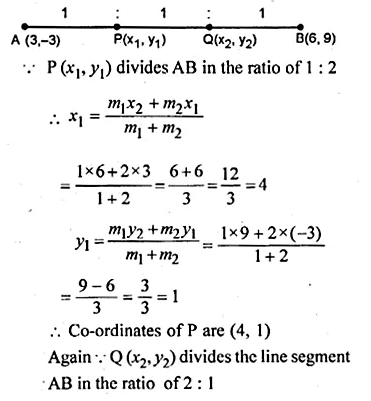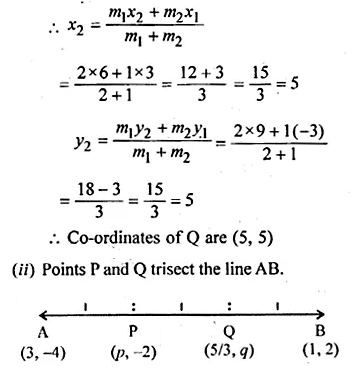Question 5.
(i) The line segment joining the points A (3, 2) and B (5, 1) is divided at the point P in the ratio 1 : 2 and it lies on the line 3x – 18y + k = 0. Find the value of k.
(ii) A point P divides the line segment joining the points A (3, – 5) and B ( – 4, 8) such that $$\frac { AP }{ PB } =\frac { k }{ 1 }$$ If P lies on the line x + y = 0, then find the value of k.
Solution:
(i) The point P (x, y) divides the line segment joining the points
A (3, 2) and B (5, 1) in the ratio 1 : 2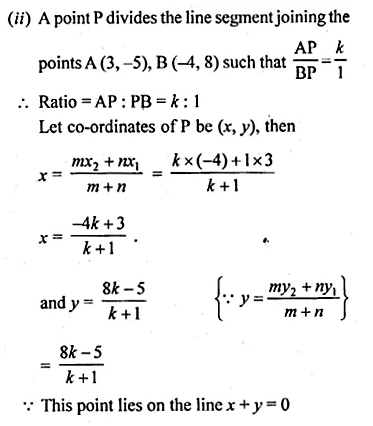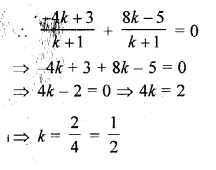Question 6.
Find the coordinates of the point which is three-fourth of the way from A (3, 1) to B ( – 2, 5).
Solution:
Let P be the required point, then
$$\frac { AP }{ AB } =\frac { 3 }{ 4 }$$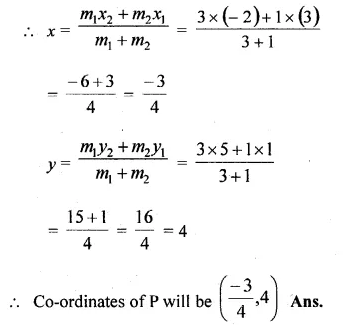Question 7.
Point P (3, – 5) is reflected to P’ in the x- axis. Also P on reflection in the y-axis is mapped as P”.
(i) Find the co-ordinates of P’ and P”.
(ii) Compute the distance P’ P”.
(iii) Find the middle point of the line segment P’ P”.
(iv) On which co-ordinate axis does the middle point of the line segment P P” lie ?
Solution:
(i) Co-ordinates of P’, the image of P (3, -5)
when reflected in x-axis will be (3, 5)
and co-ordinates of P”, the image of P (3, -5)
when reflected in y-axis will be (-3, -5)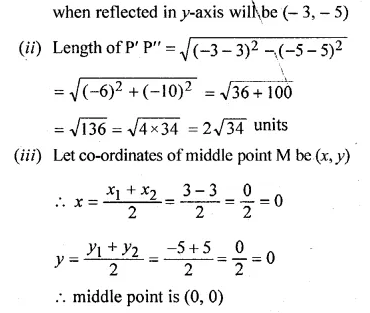Question 8.
Use graph paper for this question. Take 1 cm = 1 unit on both axes. Plot the points A(3, 0) and B(0, 4).
(i) Write down the co-ordinates of A1, the reflection of A in the y-axis.
(ii) Write down the co-ordinates of B1, the reflection of B in the x-axis.
(iii) Assign.the special name to the quadrilateral ABA1B1.
(iv) If C is the mid point is AB. Write down the co-ordinates of the point C1, the reflection of C in the origin.
(v) Assign the special name to quadrilateral ABC1B1.
Solution:
Two points A (3, 0) and B (0,4) have been plotted on the graph.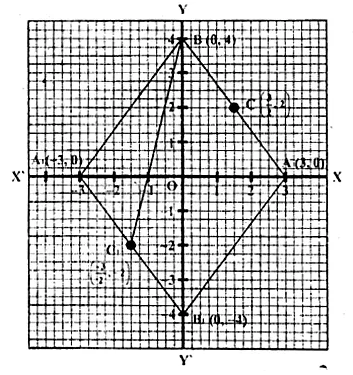(i)∵ A1 is the reflection of A (3, 0) in the v-axis Its co-ordinates will be ( -3, 0)
(ii)∵ B1 is the reflection of B (0, 4) in the .x-axis co-ordinates of B, will be (0, -4)
(iii) The so formed figure ABA1B1 is a rhombus.
(iv) C is the mid point of AB co-ordinates of C” will be $$\frac { AP }{ AB } =\frac { 3 }{ 4 }$$
∵ C, is the reflection of C in the origin
co-ordinates of C, will be $$\left( \frac { -3 }{ 2 } ,-2 \right)$$
(v) The name of quadrilateral ABC1B1 is a trapezium because AB is parallel to B1C1.

Question 9.
The line segment joining A ( – 3, 1) and B (5, – 4) is a diameter of a circle whose centre is C. find the co-ordinates of the point C. (1990)
Solution:
∵ C is the centre of the circle and AB is the diameter
C is the midpoint of AB.
Let co-ordinates of C (x, y)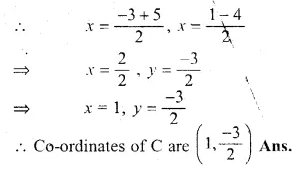Question 10.
The mid-point of the line segment joining the points (3m, 6) and ( – 4, 3n) is (1, 2m – 1). Find the values of m and n.
Solution:
Let the mid-point of the line segment joining two points
A(3m, 6) and (-4, 3n) is P( 1, 2m – 1)Question 11.
The co-ordinates of the mid-point of the line segment PQ are (1, – 2). The co-ordinates of P are ( – 3, 2). Find the co-ordinates of Q.(1992)
Solution:
Let the co-ordinates of Q be (x, y)
co-ordinates of P are (-3, 2) and mid-point of PQ are (1, -2) then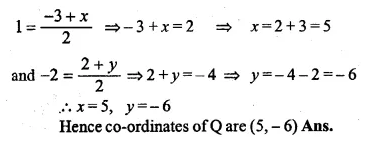Question 12.
AB is a diameter of a circle with centre C ( – 2, 5). If point A is (3, – 7). Find:
(i) the length of radius AC.
(ii) the coordinates of B.
Solution:
AC = $$\sqrt { { \left( 3+2 \right) }^{ 2 }+{ \left( -7-5 \right) }^{ 2 } }$$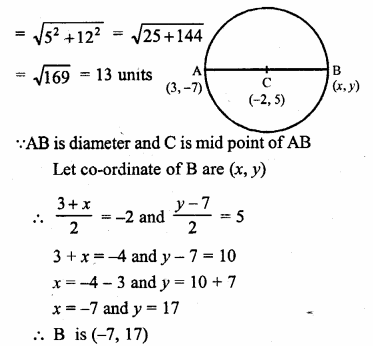Question 13.
Find the reflection (image) of the point (5, – 3) in the point ( – 1, 3).
Solution:
Let the co-ordinates of the images of the point A (5, -3) be
A1 (x, y) in the point (-1, 3) then
the point (-1, 3) will be the midpoint of AA1.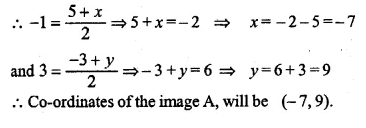Question 14.
The line segment joining A $$\left( -1,\frac { 5 }{ 3 } \right)$$ the points B (a, 5) is divided in the ratio 1 : 3 at P, the point where the line segment AB intersects y-axis. Calculate
(i) the value of a
(ii) the co-ordinates of P. (1994)
Solution:
Let P (x, y) divides the line segment joining
the points $$\left( -1,\frac { 5 }{ 3 } \right)$$, B(a, 5) in the ratio 1 : 3Question 15.
The point P ( – 4, 1) divides the line segment joining the points A (2, – 2) and B in the ratio of 3 : 5. Find the point B.
Solution:
Let the co-ordinates of B be (x, y)
Co-ordinates of A (2, -2) and point P (-4, 1)
divides AB in the ratio of 3 : 5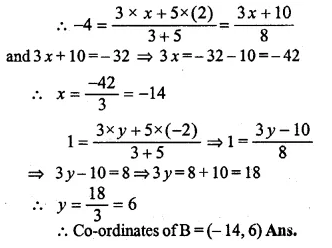Question 16.
(i) In what ratio does the point (5, 4) divide the line segment joining the points (2, 1) and (7 ,6) ?
(ii) In what ratio does the point ( – 4, b) divide the line segment joining the points P (2, – 2), Q ( – 14, 6) ? Hence find the value of b.
Solution:
(i) Let the ratio be m1 : m2 that the point (5, 4) divides
the line segment joining the points (2, 1), (7, 6).
$$5=\frac { { m }_{ 1 }\times 7+{ m }_{ 2 }\times 2 }{ { m }_{ 1 }+{ m }_{ 2 } }$$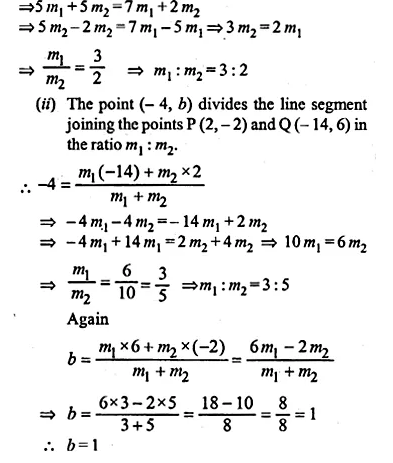Question 17.
The line segment joining A (2, 3) and B (6, – 5) is intercepted by the x-axis at the point K. Write the ordinate of the point k. Hence, find the ratio in which K divides AB. Also, find the coordinates of the point K.
Solution:
Let the co-ordinates of K be (x, 0) as it intersects x-axis.
Let point K divides the line segment joining the points
A (2, 3) and B (6, -5) in the ratio m1 : m2.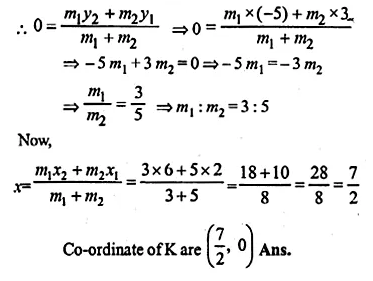Question 18.
If A ( – 4, 3) and B (8, – 6), (i) find the length of AB.
(ii) in what ratio is the line joining AB, divided by the x-axis? (2008)
Solution:
Given A (-4, 3), B (8, -6)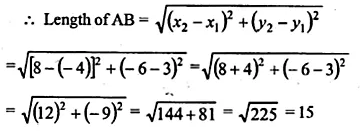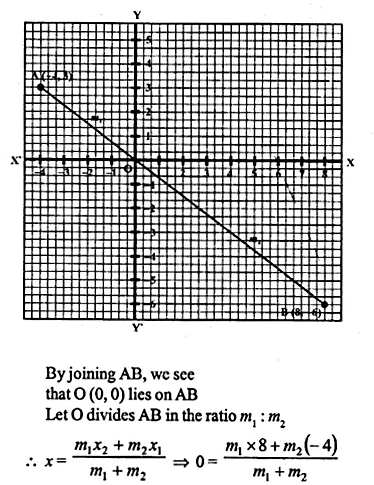Question 19.
(i) Calculate the ratio in which the line segment joining (3, 4) and( – 2, 1) is divided by the y-axis.
(ii) In what ratio does the line x – y – 2 = 0 divide the line segment joining the points (3, – 1) and (8, 9)? Also, find the coordinates of the point of division.
Solution:
(i) Let the point P divides the line segment joining the points
A (3, 4) and B (-2, 3) in the ratio of m1 : m2 and
let the co-ordinates of P be (0, y) as it intersects the y-axis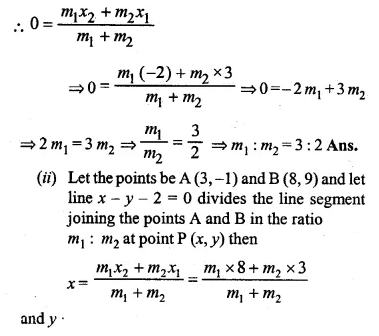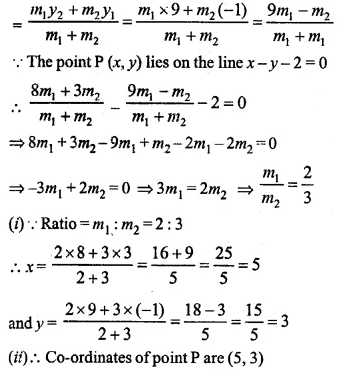Question 20.
Given a line segment AB joining the points A ( – 4, 6) and B (8, – 3). Find:
(i) the ratio in which AB is divided by the y-axis.
(ii) find the coordinates of the point of intersection.
(iii)the length of AB.
Solution:
(i) Let the y-axis divide AB in the ratio m : 1. So,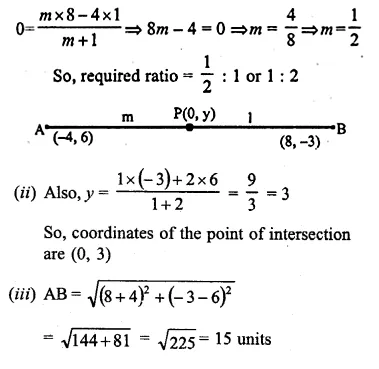Question 21.
(i) Write down the co-ordinates of the point P that divides the line joining A ( – 4, 1) and B (17,10) in the ratio 1 : 2.
(ii)Calculate the distance OP where O is the origin.
(iii)In what ratio does the y-axis divide the line AB ?
Solution:
(i) Let co-ordinate of P be (x, y) which divides the line segment joining the points
A ( -4, 1) and B(17, 10) in the ratio of 1 : 2.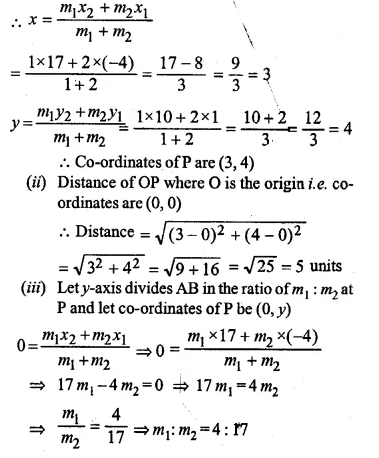Question 22.
Calculate the length of the median through the vertex A of the triangle ABC with vertices A (7, – 3), B (5, 3) and C (3, – 1)
Solution:
Let D (x, y) be the median of ΔABC through A to BC.
∴ D will be the midpoint of BC
∴ Co-ordinates of D will be,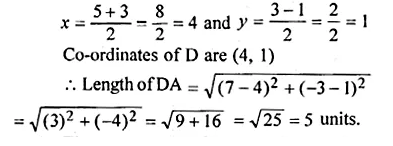Question 23.
Three consecutive vertices of a parallelogram ABCD are A (1, 2), B (1, 0) and C (4, 0). Find the fourth vertex D.
Solution:
Let O in the mid-point of AC the diagonal of ABCD
∴ Co-ordinates of O will be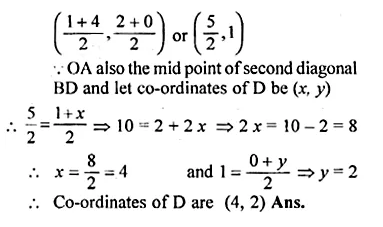Question 24.
If the points A ( – 2, – 1), B (1, 0), C (p, 3) and D (1, q) from a parallelogram ABCD, find the values of p and q.
Solution:
A (-2, -1), B (1, 0), C (p, 3) and D (1, q)
are the vertices of a parallelogram ABCD
∴ Diagonal AC and BD bisect each other at O
O is the midpoint of AC as well as BD
Let co-ordinates of O be (x, y)
When O is mid-point of AC, thenQuestion 25.
If two vertices of a parallelogram are (3, 2) ( – 1, 0) and its diagonals meet at (2, – 5), find the other two vertices of the parallelogram.
Solution:
Two vertices of a ||gm ABCD are A (3, 2), B (-1, 0)
and point of intersection of its diagonals is P (2, -5)
P is mid-point of AC and BD.
Let co-ordinates of C be (x, y), then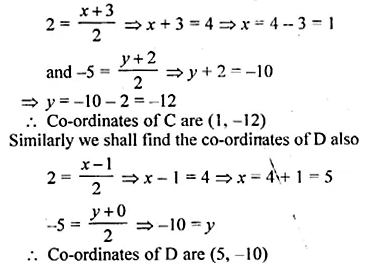Question 26.
Prove that the points A ( – 5, 4), B ( – 1, – 2) and C (5, 2) are the vertices of an isosceles right angled triangle. Find the co-ordinates of D so that ABCD is a square.
Solution:
Points A (-5, 4), B (-1, -2) and C (5, 2) are given.
If these are vertices of an isosceles triangle ABC then
AB = BC.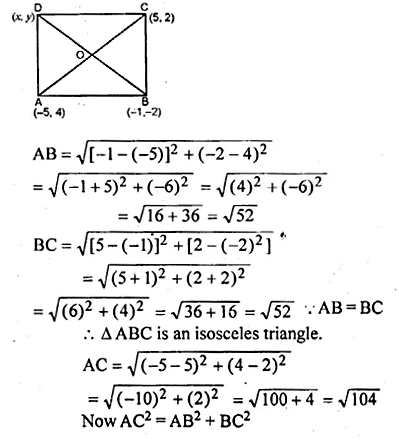Question 27.
Find the third vertex of a triangle if its two vertices are ( – 1, 4) and (5, 2) and mid point of one sides is (0, 3).
Solution:
Let A (-1, 4) and B (5, 2) be the two points and let D (0, 3)
be its the midpoint of AC and co-ordinates of C be (x, y).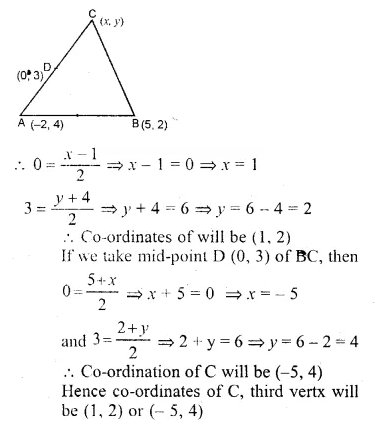Question 28.
Find the coordinates of the vertices of the triangle the middle points of whose sides are $$\left( 0,\frac { 1 }{ 2 } \right) ,\left( \frac { 1 }{ 2 } ,\frac { 1 }{ 2 } \right) and\left( \frac { 1 }{ 2 } ,0 \right)$$
Solution:
Let ABC be a ∆ in which $$D\left( 0,\frac { 1 }{ 2 } \right) ,E\left( \frac { 1 }{ 2 } ,\frac { 1 }{ 2 } \right) andF\left( \frac { 1 }{ 2 } ,0 \right)$$,
the mid-points of sides AB, BC and CA respectively.
Let co-ordinates of A be (x1, y1), B (x2, y2), C (x3, y3)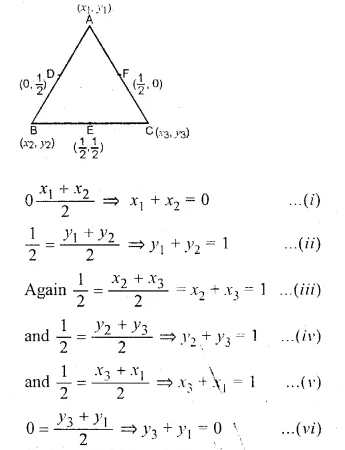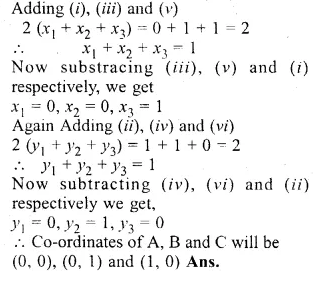Question 29.
Show by section formula that the points (3, – 2), (5, 2) and (8, 8) are collinear.
Solution:
Let the point (5, 2) divides the line joining the points (3, -2) and (8, 8)
in the ratio of m1 : m2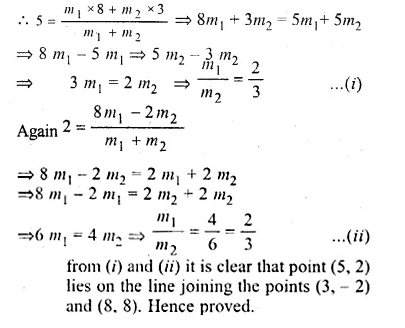Question 30.
Find the value of p for which the points ( – 5, 1), (1, p) and (4, – 2) are collinear.
Solution:
Let points A (-5, 1), B (1, p) and C (4, -2)
are collinear and let point A (-5, 1) divides
BC in the ratio in m1 : m2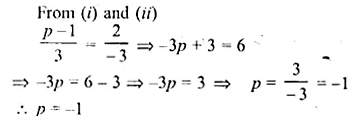Question 31.
A (10, 5), B (6, – 3) and C (2, 1) are the vertices of triangle ABC. L is the mid point of AB, M is the mid-point of AC. Write down the co-ordinates of L and M. Show that LM = $$\\ \frac { 1 }{ 2 }$$ BC.
Solution:
Co-ordinates of L will be
$$\left( \frac { 10+6 }{ 2 } ,\frac { 5-3 }{ 2 } \right) or\left( \frac { 16 }{ 2 } ,\frac { 2 }{ 2 } \right) or(8,1)$$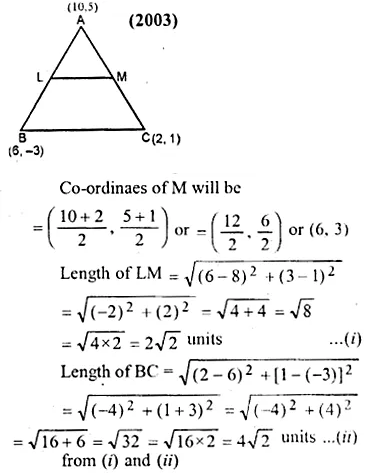Question 32.
A (2, 5), B ( – 1, 2) and C (5, 8) are the vertices of a triangle ABC. P and.Q are points on AB and AC respectively such that AP : PB = AQ : QC = 1 : 2.
(i) Find the co-ordinates of P and Q.
(ii) Show that PQ = $$\\ \frac { 1 }{ 3 }$$ BC.
Solution:
A (2, 5), B (-1, 2) and C (5, 8) are the vertices of a ∆ABC,
P and Q are points on AB
and AC respectively such that $$\frac { AP }{ PB } =\frac { AQ }{ QC } =\frac { 1 }{ 2 }$$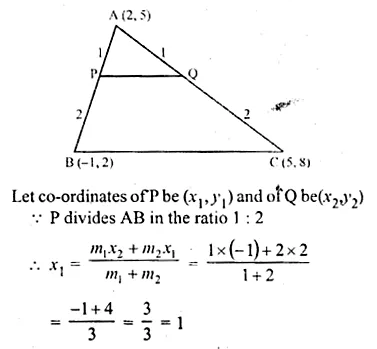Question 33.
The mid-point of the line segment AB shown in the adjoining diagram is (4, – 3). Write down die co-ordinates of A and B.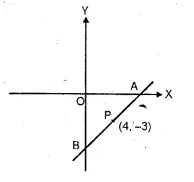Solution:
A lies on x-axis and B on the y-axis.
Let co-ordinates of A be (x, 0) and of B be (0, y)
P (4, -3) is the mid-point of ABQuestion 34.
Find the co-ordinates of the centroid of a triangle whose vertices are A ( – 1, 3), B(1, – 1) and C (5, 1) (2006)
Solution:
Co-ordinates of the centroid of a triangle,
whose vertices are (x1, y1), (x2, y2) andQuestion 35.
Two vertices of a triangle are (3, – 5) and ( – 7, 4). Find the third vertex given that the centroid is (2, – 1).
Solution:
Let the co-ordinates of third vertices be (x, y)
and other two vertices are (3, -5) and (-7, 4)
and centroid = (2, -1).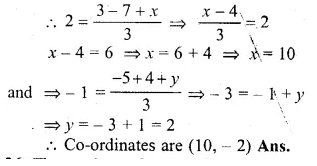Question 36.
The vertices of a triangle are A ( – 5, 3), B (p – 1) and C (6, q). Find the values of p and q if the centroid of the triangle ABC is the point (1, – 1).
Solution:
The vertices of ∆ABC are A (-5, 3), B (p, -1), C (6, q)
and the centroid of ∆ABC is O (1, -1)
co-ordinates of the centroid of ∆ABC will be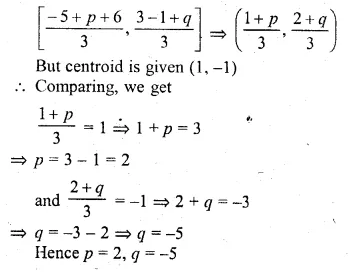Hope given ML Aggarwal Class 10 Solutions for ICSE Maths Chapter 11 Section Formula Ex 11 are helpful to complete your math homework.

If you have any doubts, please comment below. Learn Insta try to provide online math tutoring for you.